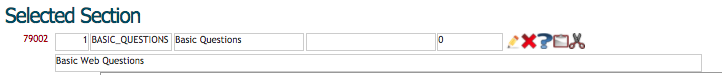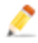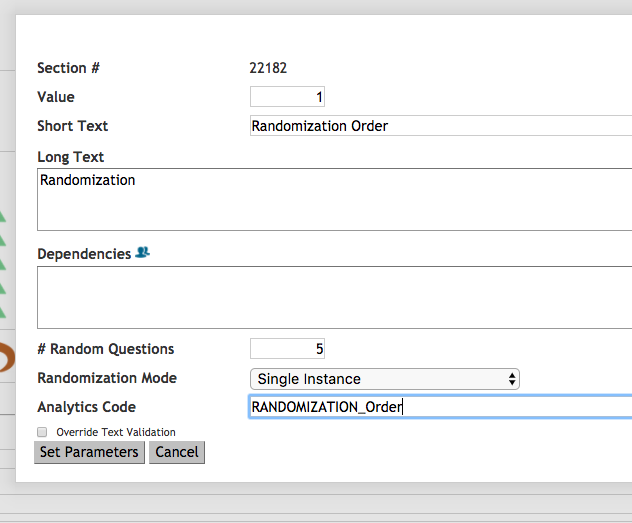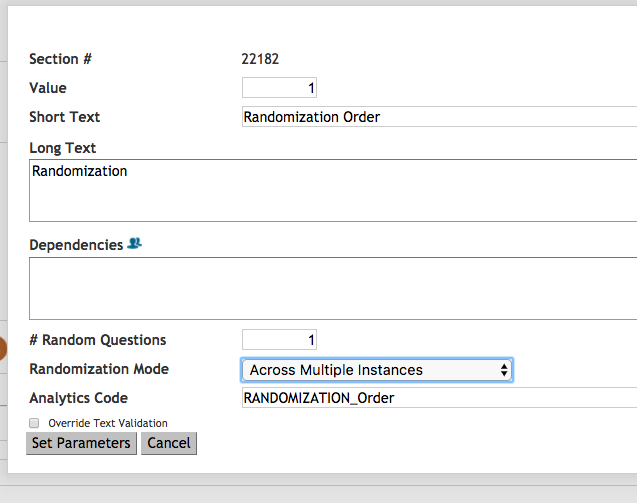mEMA allows you to randomize question presentation in three ways:

1. Randomly present survey elements (Questions and Sections) within a single survey instance: You can select a sub-set of Sections or Questions from the total pool to be presented at each instance to each user.
2. Randomize the presentation order of Questions or Sections within a single survey instance: You can select how many Questions in a Section or how many Sections in a Form will be presented at each new instance in addition to the order they will be presented.
3. Randomly present survey elements across survey instances: You can select a sub-set of Sections or Questions from the total pool to be presented one per instance across all possible instance in an assignment.

Note: This feature should not be used in combination with branching logic such as dependencies

1. Randomly present survey elements (Questions and Sections) within a single survey instance

You can set the system to present a randomly selected subset of the Questions in a Section or a subset of the Sections in a Form at each new survey instanceTo present a random subset of Questions in any Section navigate to the Section and click the pencil icon EditThis window will appear:Enter the number of questions you would like to show in the field "# Randomize Questions". The number must be lower than the total number of questions in the section.

Example: If there are 10 questions in the section and you want only 5 of them to be presented at each survey instance, enter "5" into the "# Random Questions" field.

Randomization Mode: Choose "Single Instance"

The app will present the participant with 5 randomly selected Questions from the total possible pool in the section. By default they are presented in order they appear in the Survey Editor.

You can do the same thing at the Form level, that is, randomly present a subset of Sections within any one survey instance. Follow the step above but in this case Edit at the Form (not the Section) level.

2. Randomize the presentation order of Questions or Sections within a single survey instance survey

You can set the system to present a randomly selected subset of the Questions in a Section or a subset of the Sections in a Form and to randomize the presentation order at each new survey instance.

Follow the steps above describing how to randomize Questions and Sections. Then add a "-" sign in front of the number you type in the "# Random Questions" field.

Example: If there are 10 questions in the Section and you want only 5 of them to be presented at each survey instance in a different order each time, enter "-5" into the "# Random Questions" field.

The number you type in the  "# Random Questions" field can be equal to or less than the total number of Questions in the Section.

Example: If there are 10 questions in the Section and you want all of them to be presented at each survey instance in a different order each time, enter "-10" into the "# Random Questions" field.

3. Randomly present survey elements across survey instances
You can set the system to present a randomly selected subset of the Questions in a Section across the course of a multi-day study. In this case the system will select one Question from the Section to be presented at each new instance of the survey during any assignment (which can last for multiple days/ weeks).

Navigate to the Section and click Edit.

This window will appear:Enter the number of questions you would like to show each survey instance in the field "# Randomize Questions".

Example: If there are 10 questions in the section and you want 1 of them to be presented at each survey instance, enter "1" into the "# Random Questions" field.

Randomization Mode: Choose "Across Multiple Instances"

The app will present the participant with 1 randomly selected Question from the total possible pool in the section at each instance.

At the next instance the system will present another of the Questions but not any that have already been presented to that user